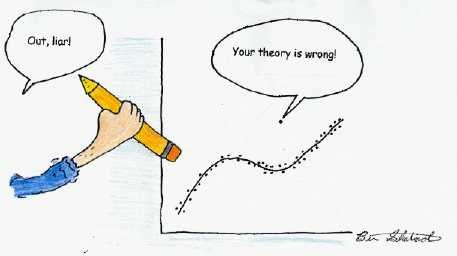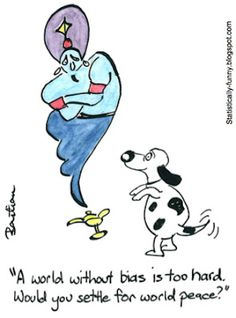## Data mining bias, a mathematical look. Part 2.

On my first post about the mathematical basis of data mining bias (DMB) we came up with a mathematical formulation for mining bias (equation 5) that is applicable to the entire space of trading systems within a given financial time series. Today we are going to use this mathematical derivation to drift further away from this ideal case with the aim to consider a more realistic case where the number of systems that we explore are a subset of the total number of possible systems within a set of real market data. By doing this we will consider problems such as the possible bias introduced by space selection and how this can be measured when performing data mining bias exercises. We will come up with mathematical equations that will allow us to develop a deep understanding of how DMB is expected to evolve as the size of the logic space (which is simply the set of mined systems) changes.In our mathematical definition of mining bias it became clear that this value is entirely dependent on just two quantities: the average number of systems of interest found on random data sets and the number of systems of interest found on the real data. Given that the formulation is independent of the space size it is therefore tempting to assume that there is no introduction of bias when the size of the logic space changes from all possible systems that could be found on a financial time series to a much smaller set. This would be true if the distribution of systems of interest within the random and real data was homogeneous through the entire logic space but the problem changes drastically when we consider the fact that systems of interest (that is systems that you would want to trade) are not distributed in this manner.

So what happens when we have a non-homogeneous distribution of trading systems? If this happens then a reduction in the size of the logic space changes bias by a reduction in both the number of systems on the real and random data sets. The number of systems in the new space therefore becomes the number of total systems available minus the number of systems that are outside the logic space we have selected (Equation 6). This new equation has some important implications to mining exercises as it introduces new variables Sout_real (the number of systems outside the selected space on the real time series) and Sour_random(i) which represents the same quantity for each random time series. It is clear that for a series where systems of interest are homogeneously distributed equation 6 is simplified to equation 5 for any given subset of systems while for a non-homogeneous case our accuracy in the determination of DMB depends fundamentally on the difference between Sout_real and Sout_random(i).

$DMB_{allSystems}= \frac{\sum_{i=1}^T{S_{random_{i}}}}{T\times S_{real}}$ (Equation 5)

$DMB_{systemSubset}= \frac{\sum_{i=1}^T{(S_{random_{i}}-Sout_{random_{i}})}}{T\times (S_{real}-Sout_{real})}$ (Equation 6)

The importance of the above becomes evident when we consider an extreme case. Suppose that our mining space changes from the universe of all possible systems to simply one strategy and that strategy happens to be a strategy of interest. If systems were distributed in the same manner on random data sets and the real data then the average frequency of that system being a system of interest on random data sets would give us an accurate idea of DMB as per equation 5. This assumption can be tested by increasing the size of the logic space, if the DMB changes as a function of the size of the logic space, then it means that the difference between Sout_real and Sout_random(i) is significant enough to negatively alter our determination of mining bias. We have a selection bias that is introduced when we select a given logic space to search for trading strategies that is not the entire set of potential strategies.

The above developments give us an important tool. We know that for our DMB determination to be accurate we do not need Sout_real and Sout_random(i) to be zero (we don’t need to look across all possible systems that can exist) but we need them to have essentially the same value. This means that we need to make sure to a very good degree that the distribution of systems is fairly homogeneous within the space that we are mining. We can test this assumption by increasing the size of our logic space to look at how our DMB changes, if the DMB increases as our logic space gets larger then we can say that we are in a case where Sout_random(i) > Sout_real and therefore we were underestimating mining bias when using that reduced space. These two variables are in essence a function of the logic space (they become 0 when the space equals the maximum number of possible systems), so the question is whether there is a size of the logic space where we can assume equation 5 to be true.To do this we can attempt an experiment in which we increase the size of the logic space systematically, plotting the DMB as a function of the logic space size (next post on the series!), which allows us to infer the nature of the DMB as a function of the logic space. If at some point the DMB becomes constant, then we can say that from that point on the logic space size becomes irrelevant. We could therefore mine spaces above that size without having to worry about Sout_random(i) and Sout_real since we can in essence consider them both approximately equal. This size, per my experiments, turns to be around 500 million systems on several financial time series I have tested (more on this on later posts).

If you would like to learn more about measuring DMB and how you too can generate systems using the power of GPU technology please consider joining Asirikuy.com, a website filled with educational videos, trading systems, development and a sound, honest and transparent approach towards automated trading.

You can leave a response, or trackback from your own site.